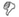## Definitions

Sorry, no definitions found. Check out and contribute to the discussion of this word!

## Etymologies

Sorry, no etymologies found.

## Examples

Sorry, no example sentences found.

Rates text on a 100-point scale; the higher the score, the easier it is to understand the document. For most standard documents, aim for a score of approximately 60 to 70.

The formula for the Flesch Reading Ease score is:

206.835 – (1.015 x ASL) – (84.6 x ASW)

where:

ASL = average sentence length (the number of words divided by the number of sentences)

ASW = average number of syllables per word (the number of syllables divided by the number of words)

- Microsoft

July 24, 2008